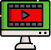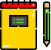VideosNotes

### FACTORS OF NUMBERS - 1

FACTORS OF NUMBERS
Factors are the numbers which divide the given number exactly, whereas the multiples are the numbers which are multiplied by the other number to get specific.
Views: N/A
Video Duration: 11:55 (Part 1 of 3)